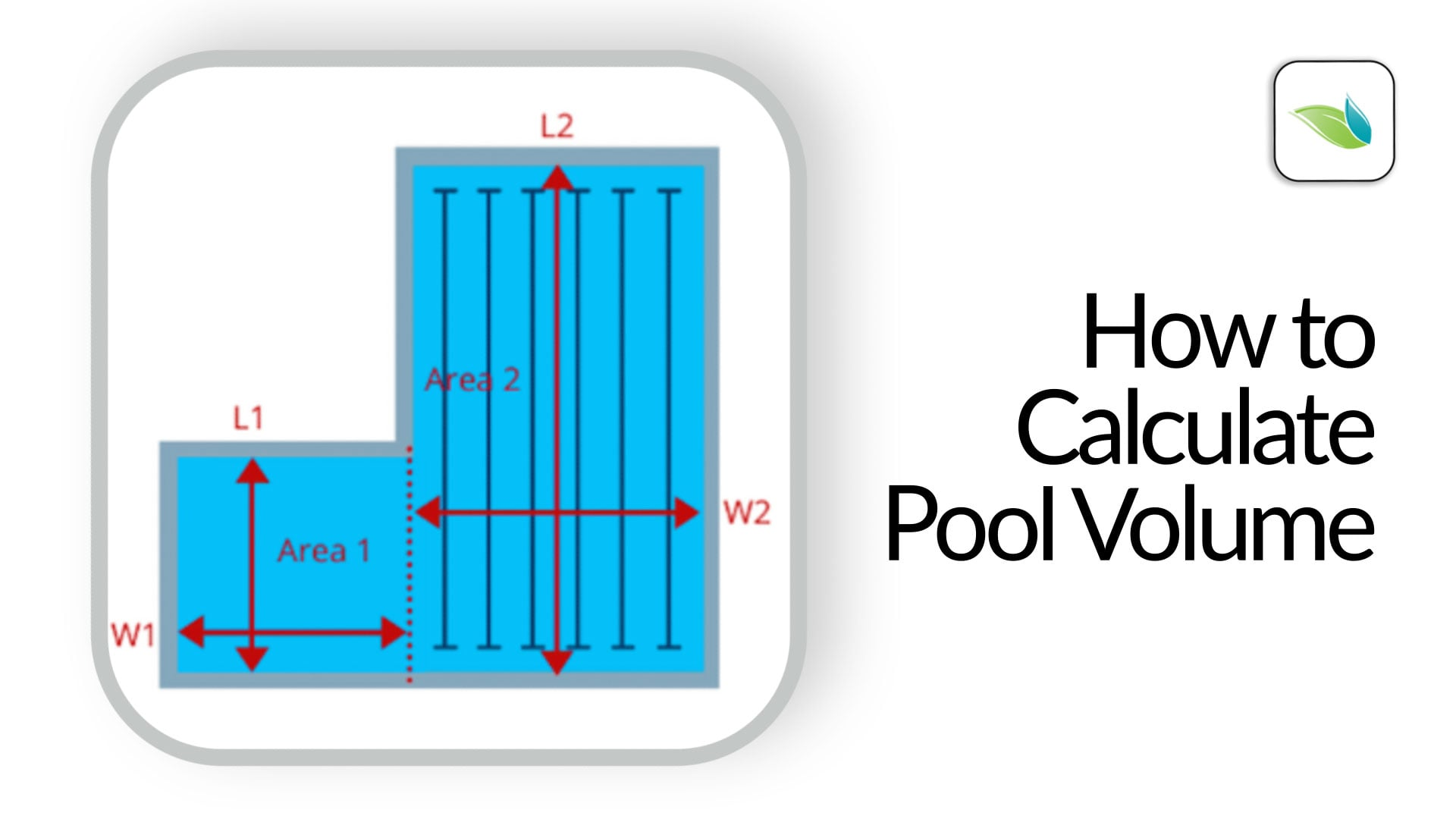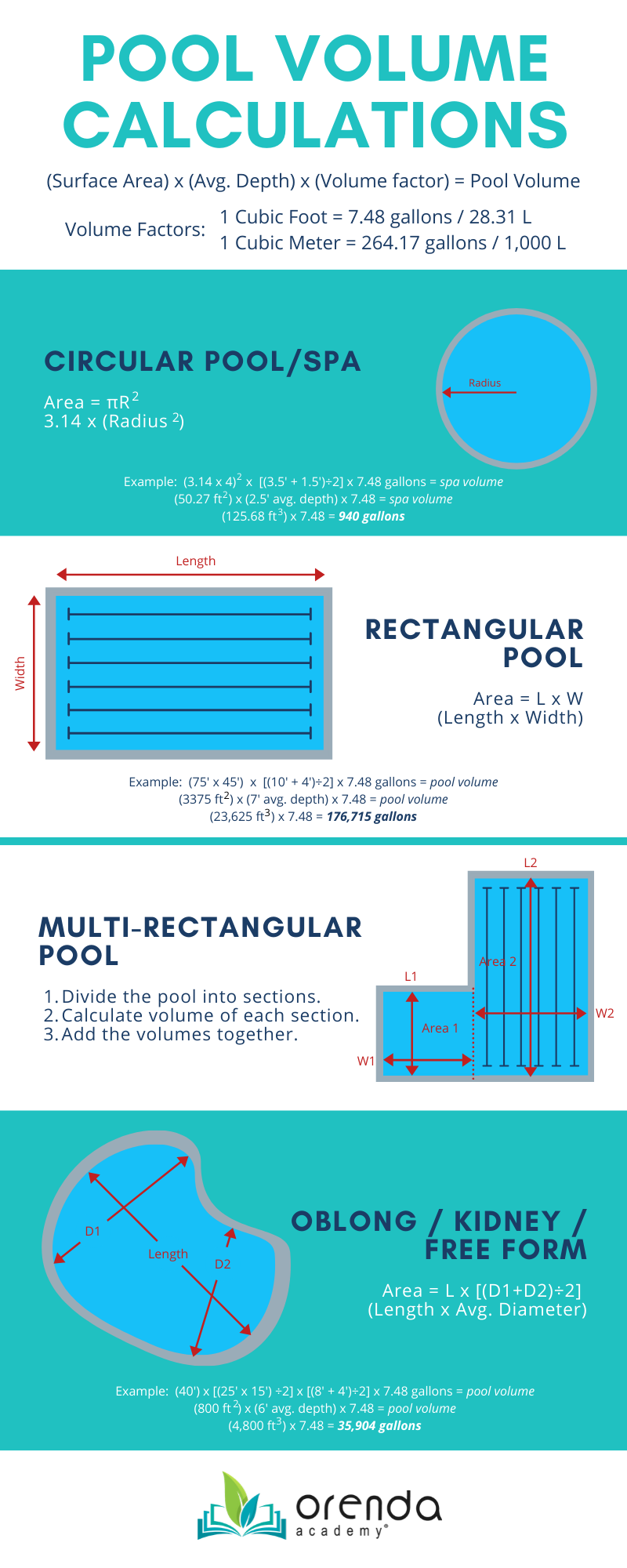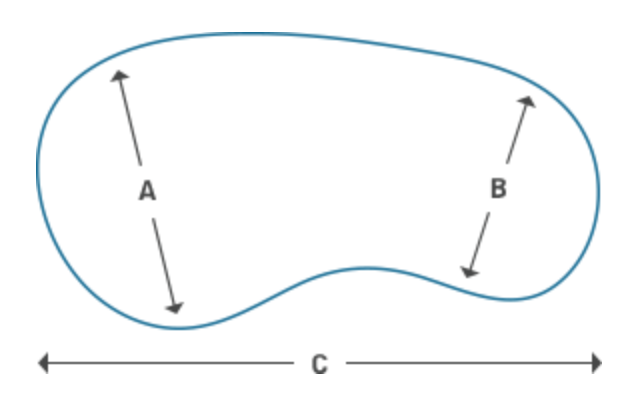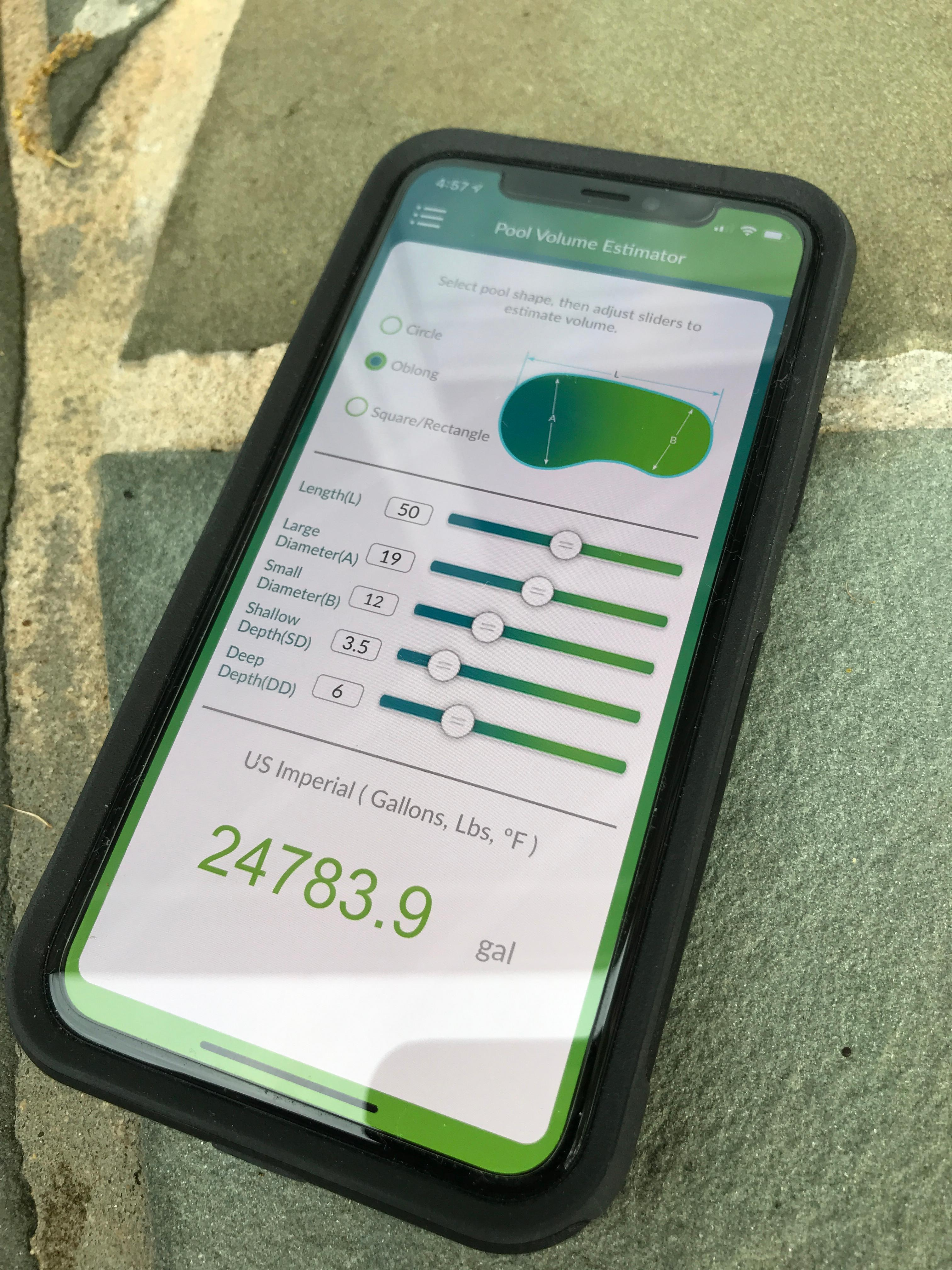# How to Calculate Swimming Pool Volume"How do I calculate the volume of a pool?" This article will guide you on how to measure a swimming pool and calculate its volume of water. Let's begin.

It is essential to know the volume of a swimming pool to make accurate chemistry adjustments. Even veteran pool professionals can eyeball a pool and estimate the volume of it...but how accurately? We have found through surveying many of our customers around the country, that pool volume estimations–without measuring–are often off by more than 2000-3000 gallons (7571 - 11,356 L). On average, that's about a 10% error. Ten percent!

That could mean 10% more chemicals used (assuming you measure chemicals properly in the first place), or it could mean under-dosing the pool every visit. Neither are good outcomes, and will lead to water chemistry challenges.

Some pools are fortunate enough to have a specific volume on record (based on the pool builder's design software). But many pools are not so fortunate. For those pools, we must estimate their volume by measuring dimensions and doing some calculations. There are several pool volume calculators available online, and our free Orenda App has a Pool Volume Estimator feature that uses the formula provided in the NSPF's Certified Pool Operator (CPO) training manual.HELPFUL IDEA: Want help measuring? You can use a laser measuring tool. Set up a bucket or test kit on the opposite side of the pool, and aim the laser at it. This is a fast and easy way to measure dimensions of your pool accurately. For depth, use your brush/net/vacuum pole, but without an attachment on it. Make note on the pole how deep the water comes up on the pole, then measure it when you pull it out of the water.

## Swimming Pool Volume CalculationsPool volume is calculated based on length, width, and average depths. Basically we are trying to figure out the cubic area of the entire swimming pool, then multiply by a given volume of water per that cubic unit of measurement. Here are the multipliers, depending on the units of measurement you use.

• 1 Cubic Foot = 7.48 gallons / 28.31 L
• 1 Cubic Meter = 264.17 gallons / 1,000 L

The infographic above (which we spent WAY too much time creating ourselves) gives you the basics for those common shapes of pools. Obviously, free form pools that are not conveniently kidney-shaped are more difficult.

### Rectangular Pools and Multi-Rectangular Pools

(Length) x (Width) x (Average Depth) x (7.48) = Pool Volume in Gallons

Average depth requires–at a minimum–measuring the deepest depth and the shallowest, and finding the average of the two. But some pools have mostly shallow and a steep drop off into a small deep area, or vice versa. In cases like that, it can be helpful to take more than two depth measurements to get a more accurate average depth measurement.

A normal rectangular pool is fairly straightforward to calculate volume. Multi-shaped pools, however, need to be calculated by dividing the pool into sections, then adding them together. For instance, an L shape pool should be divided into two rectangles, then add the two volumes of water together.

### Circular Pools and Spas

(Surface Area) x (Average Depth) x (7.48) = Pool/Spa Volume in Gallons

If you know the diameter or radius of the circle, you can calculate the area with the help of Pi (π). The formula is πR2, or [3.14 x (Radius squared)]. After you know the area, all you need is to know the average depth. Spas are usually pretty easy. Measure the depth of the bench, and the depth of the floor. The average depth is [(floor depth + bench depth)÷2].  Then just multiply that cubic area by the volume factor (7.48 for gallons). Above ground pools are even easier, because they only have one depth throughout.

### Free Form / Oblong / Kidney-shaped Pools

(Length) x (Approximate Width) x (Average Depth) x (7.48) = Pool Volume in Gallons

Free form pools are more and more popular these days. Unfortunately they are also more difficult to measure the volume on. If you have a free-form pool, contact the pool builder, and hope they saved the volume of the pool from the design software they used. Otherwise, you're going to have to do the best you can with dimensions you can measure.The general idea is to try to get the area as accurately as possible, then multiply by average depth, and again by the volume factor (7.48 for gallons). To get area on a kidney shaped pool, Measure diameters across each of the main round areas. Add them together and find the average diameter, which will function as your approximate width. In the diagram here, A and B represent two diameters across the pool.

[(A + B)÷2] = Average Diameter = Approximate Width

Then simply multiply the "width" by the length to find your area. Take your area, multiply by average depth and the volume factor, and you have an approximate pool volume.

Alternatively, if you have the time and the patience, there is a chemical way to measure pool volume within a very small margin of error. It involves diluted water samples and alkalinity testing.

## The Orenda App

If you already use the Orenda App, you know we have a Pool Volume Estimator built into it. We continue to update and improve upon it, so it will look different as we continue to improve it. But the formulas do not change. Currently we offer volume estimations for Rectangular, Circular and Oblong/Kidney pool shapes.

Whatever method you use to calculate pool volume, it is important to do so. Not measuring pool volume is one of the most common bad habits we see that cause problems and wasted money.